# Finite state machine (FSM) counter design: Gray codes have a useful property in that consecutive numbers differ in only a single bit position. Table 1 lists a 3-bit modulo 8 Gray code representing the...

Finite state machine (FSM) counter design: Gray codes have a useful property in that consecutive numbers differ in only a single bit position. Table 1 lists a 3-bit modulo 8 Gray code representing the numbers 0 to 7. Design a 3-bit modulo 8 Gray code counter FSM.

a) First design and sketch a 3-bit modulo 8 Gray code counter FSM with no inputs and three outputs, the 3-bit signal Q2:0. (A modulo N counter counts from 0 to N − 1, then repeats. For example, a watch uses a modulo 60 counter for the minutes and seconds that counts from 0 to 59.) When reset, the output should be 000. On each clock edge, the output should advance to the next Gray code. After reaching 100, it should repeat with 000.

Clearly show your black box, state transition diagram, next state and output tables, your state encodings, next state and output equations, and circuit diagram.

b) Now add an UP input. If UP = 1, the counter advances to the next number (i.e., from 000→001, 001→011, etc.). If UP = 0, the counter retreats to the previous number (i.e., from 000→100, 100→101, 101→111, etc.). Go through all the steps of FSM design (1. Identify inputs and outputs 2. Sketch state transition diagram 3. Write state transition table 4. Select state encodings 5. Rewrite state transition table with state encodings 6. Write output table 7. Write Boolean equations for next state and output logic 8. Sketch the circuit schematic). Clearly label each part and finish with drawing the circuit that implements the FSM.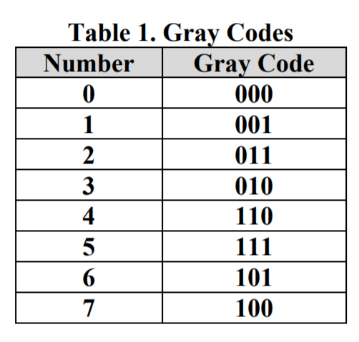Table 1. Gray Codes Number Gray Code 001 011 010 110 2 4 101 100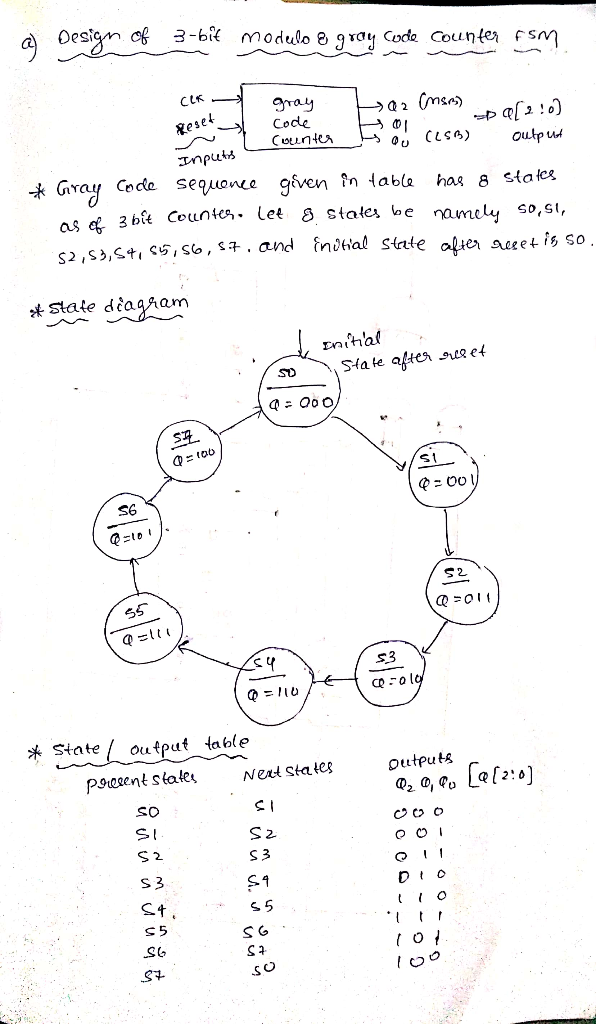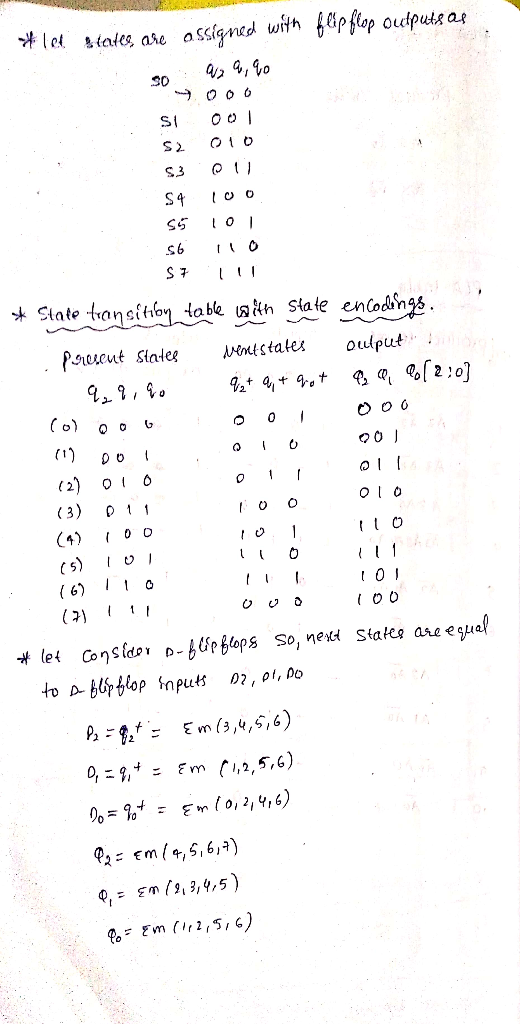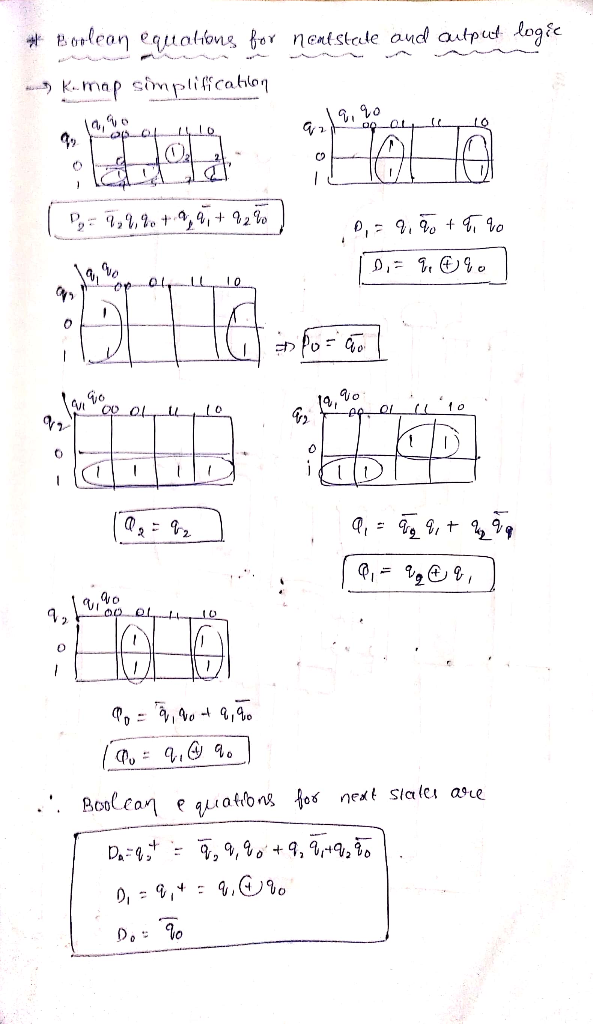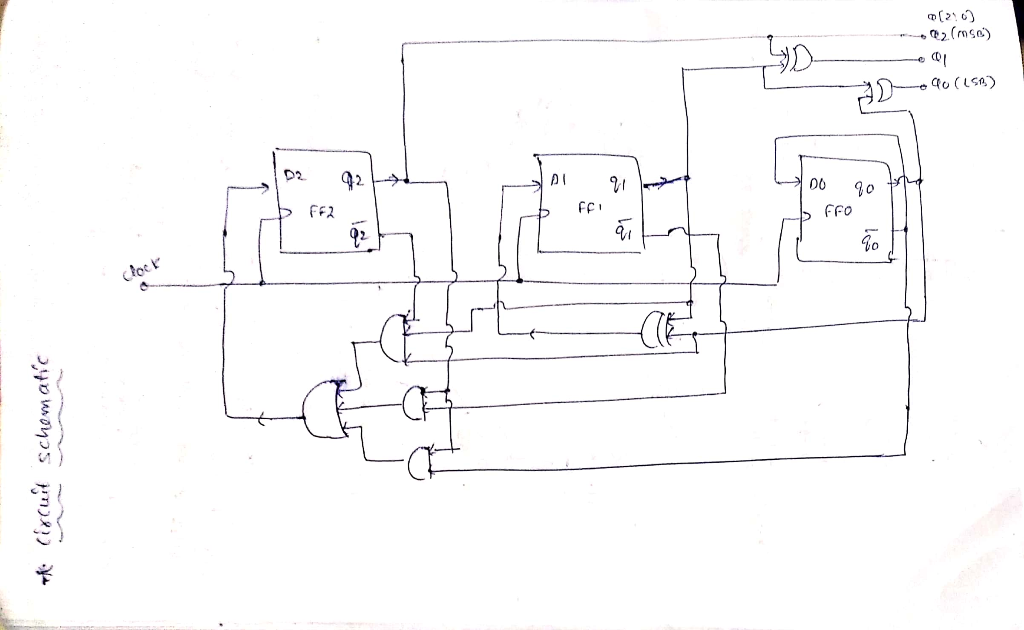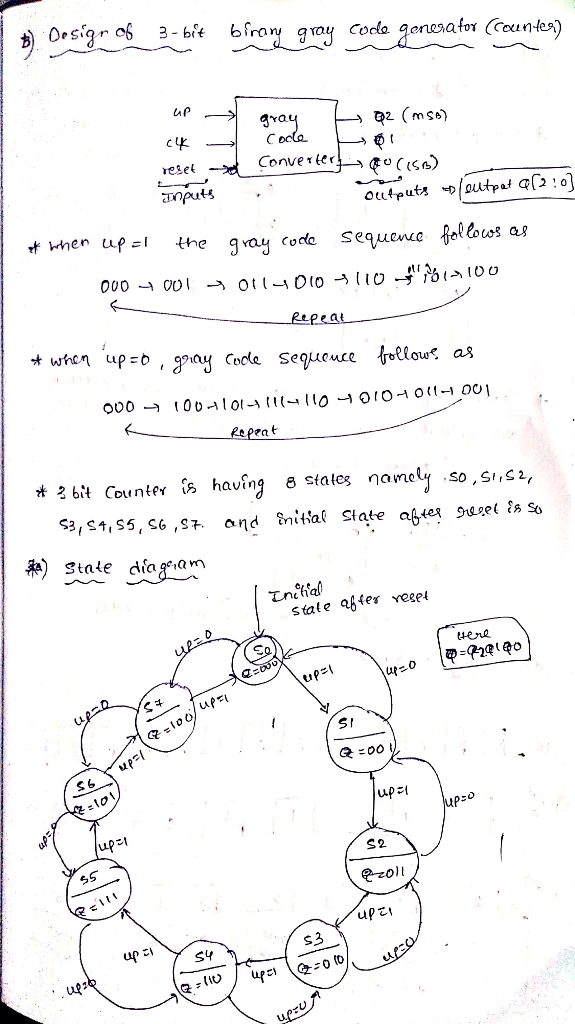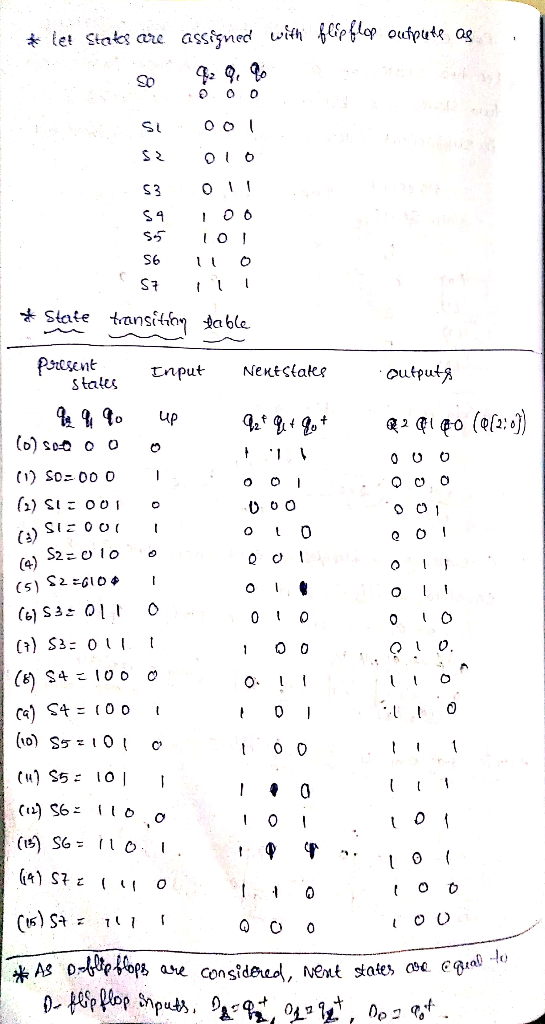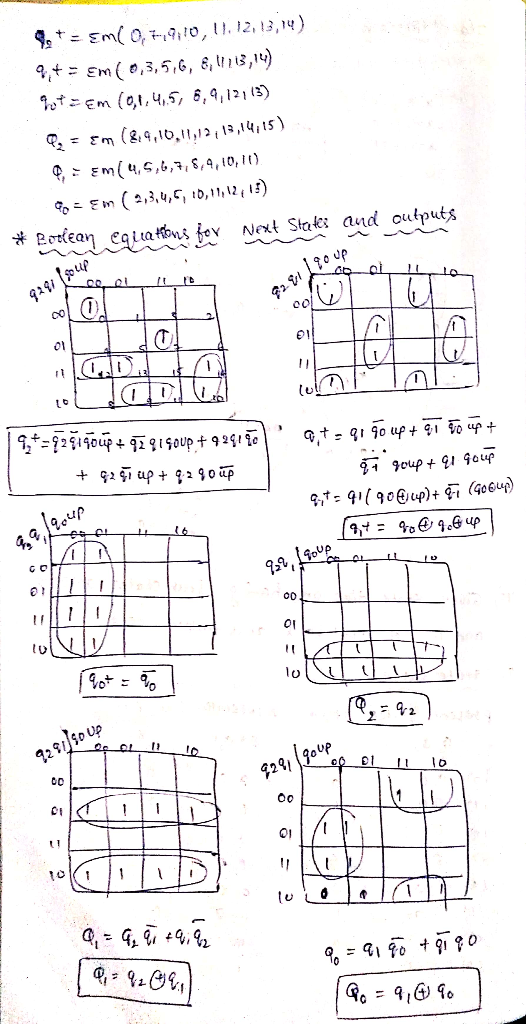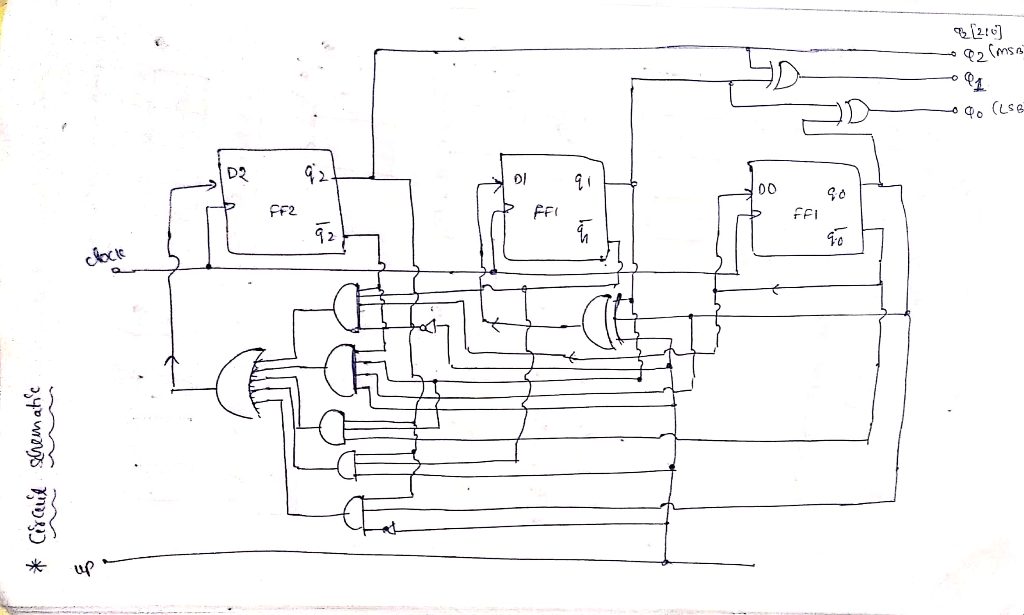##### Add Answer of: Finite state machine (FSM) counter design: Gray codes have a useful property in that consecutive numbers differ in only a single bit position. Table 1 lists a 3-bit modulo 8 Gray code representing the...
Similar Homework Help Questions
• ### Question 4 State Machines (25 marks) a. (5 marks) A 3-bit Gray code counter advances on...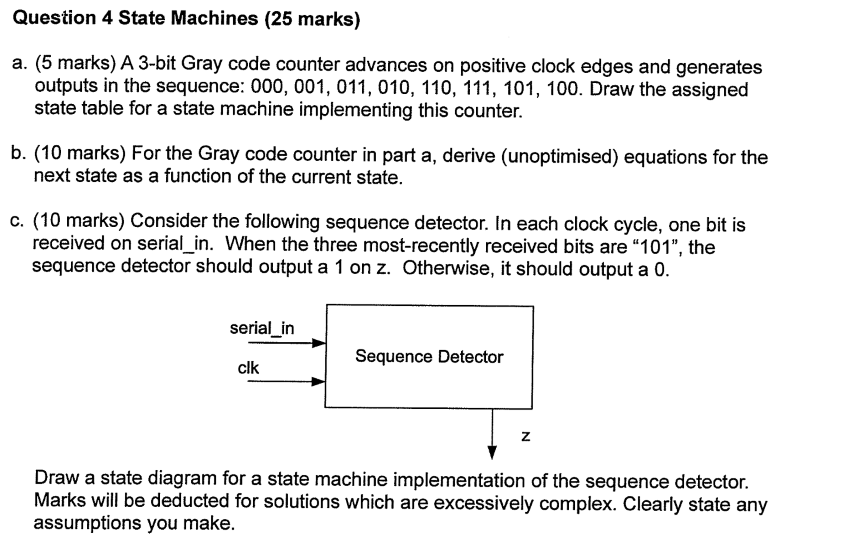Question 4 State Machines (25 marks) a. (5 marks) A 3-bit Gray code counter advances on positive clock edges and generates outputs in the sequence: 000, 001, 011, 010, 110, 111, 101, 100. Draw the assigned state table for a state machine implementing this counter. b. (10 marks) For the Gray code counter in part a, derive (unoptimised) equations for the next state as a function of the current state. c. (10 marks) Consider the following sequence detector. In each...

• ### Design a Verilog model that describes the following state diagram. (Test bench and simulation are not required) 1. 01 10 1- 10 10 01 01 10 or 01) 01 Design a Verilog model that describes a synchr...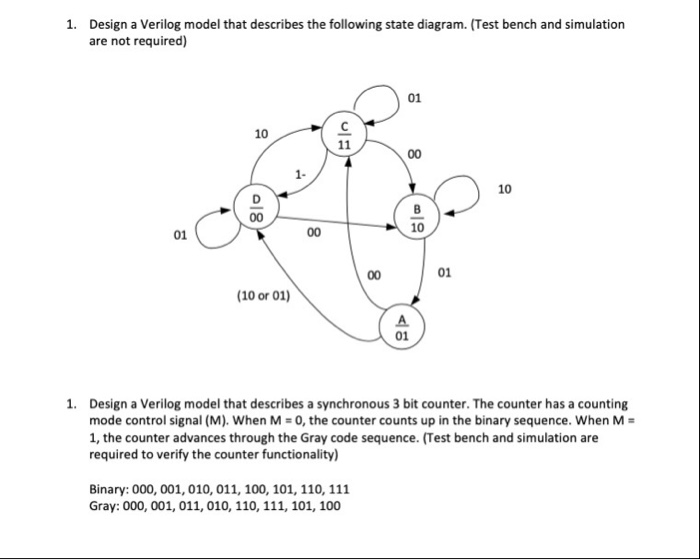Design a Verilog model that describes the following state diagram. (Test bench and simulation are not required) 1. 01 10 1- 10 10 01 01 10 or 01) 01 Design a Verilog model that describes a synchronous 3 bit counter. The counter has a counting mode control signal (M), when M-o, the counter counts up in the binary sequence, when M- 1, the counter advances through the Gray code sequence. (Test bench and simulation are required to verify the counter...

• ### Digital Logic Design: counter problem

Design a 3-bitsynchronous counter that produces the sequence000, 010, 011, 001, 101, 100, 000, etc. (using only NAND gates and D flip flops)whenever the single input is 1. The counterretains its current value whenever the input bitis 0. The output should only change on therising edge of the clock.What would the circuit diagram for this look like

• ### please answer question 4 (all parts of question4 please) will rate! 3. (30 pts) Design a 2-bit Gray code generator that ropetitively delivers the sequence 00301911-10-00when the input signal UP-...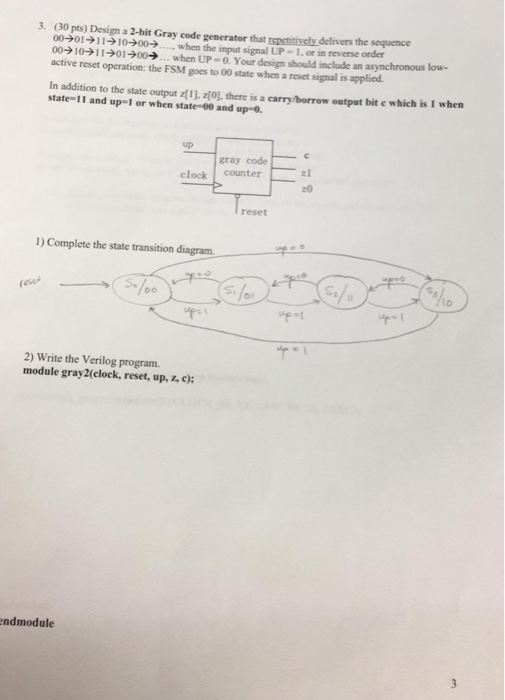please answer question 4 (all parts of question4 please) will rate! 3. (30 pts) Design a 2-bit Gray code generator that ropetitively delivers the sequence 00301911-10-00when the input signal UP- 1,or in reverse order 009 10기け01 →00→ when UP-0. Your design should include an asynchronous low. active reset operation: the FSM goes to 00 state when a reset signal is applied In addition to the state output z[1). 2. there is a carry/borrow output bit e which is I when...

• ### Design a system whose output goes high only after 8 consecutive 1's appear on the input;...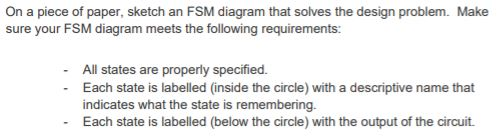Design a system whose output goes high only after 8 consecutive 1's appear on the input; once the output goes high, it takes four consecutive 0's on the input to make the output go low again. You will use one switch as the input, and one button as the clock. Assign a binary state code to each state of your FSM. On a piece of paper, develop a truth table for the next state and output logic. On a piece...

• ### Exercise 5.6: Generic Binary-to-Gray Converter The regular binary code, which consists of code words ordered according...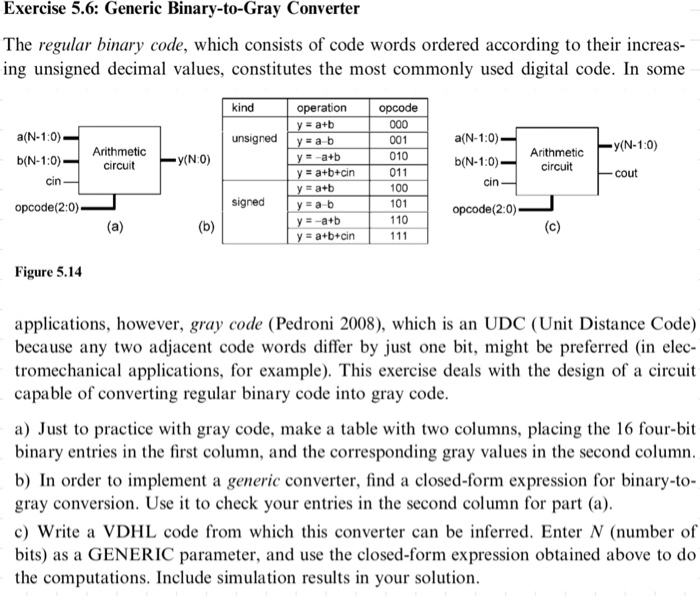Exercise 5.6: Generic Binary-to-Gray Converter The regular binary code, which consists of code words ordered according to their increas ing unsigned decimal values, constitutes the most commonly used digital code. In some kind operation opcode 000 ya+b a(N-1:0) b(N-1:0) a(N-1:0) unsigned ya b 001 y(N- 1:0) Arithmetic Arithmetic 010 y-a+b ya+b+cin y(N:0) b(N-1:0 circuit circuit 011 cout cin cin 100 ya+b signed 101 ya b opcode(2:0) opcode(2:0) 110 y-a+b (a) (b) (c) 111 ya+b+cin Figure 5.14 applications, however, gray code...

• ### 9. Product State Graph ou are asked to design a sequence detector to detect the input codes 10 and 01. The input of the circuit is and the output is Z, which only changes at a clock edge. Overla...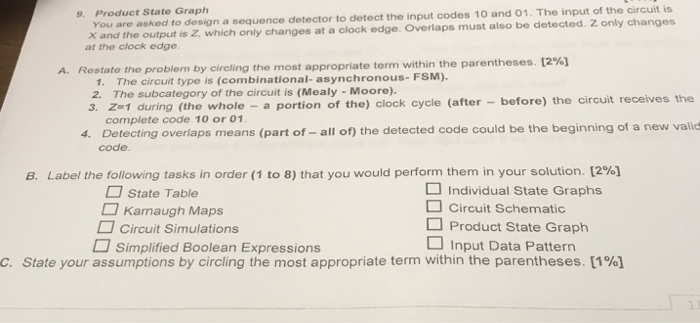9. Product State Graph ou are asked to design a sequence detector to detect the input codes 10 and 01. The input of the circuit is and the output is Z, which only changes at a clock edge. Overlaps must also be detected. Z only changes at the clock edge . [2%] A. Restate the problem by circling the most appropriate term within the parentheses 1. The circuilt type is (combinational- asynchronous- FSM). 2. The subcategory of the circuit is...

• ### Use a behavioral Verilog model to design a 3-bit fault tolerant up-down counter. For each flip-fl...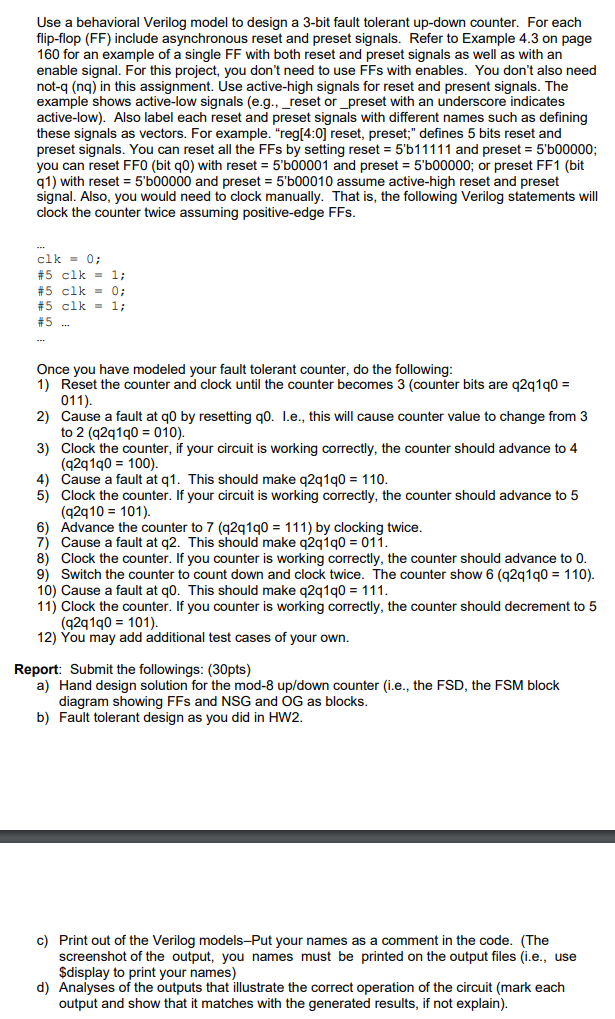Use a behavioral Verilog model to design a 3-bit fault tolerant up-down counter. For each flip-flop (FF) include asynchronous reset and preset signals. Refer to Example 4.3 on page 160 for an example of a single FF with both reset and preset signals as well as with an enable signal. For this project, you don't need to use FFs with enables. You don't also need not-q (nq) in this assignment. Use active-high signals for reset and present signals. The example...

• ### how to slove 4-25,26,27 ?? and please 2way slove state assignment gray code and counting Order or tIne Circuit....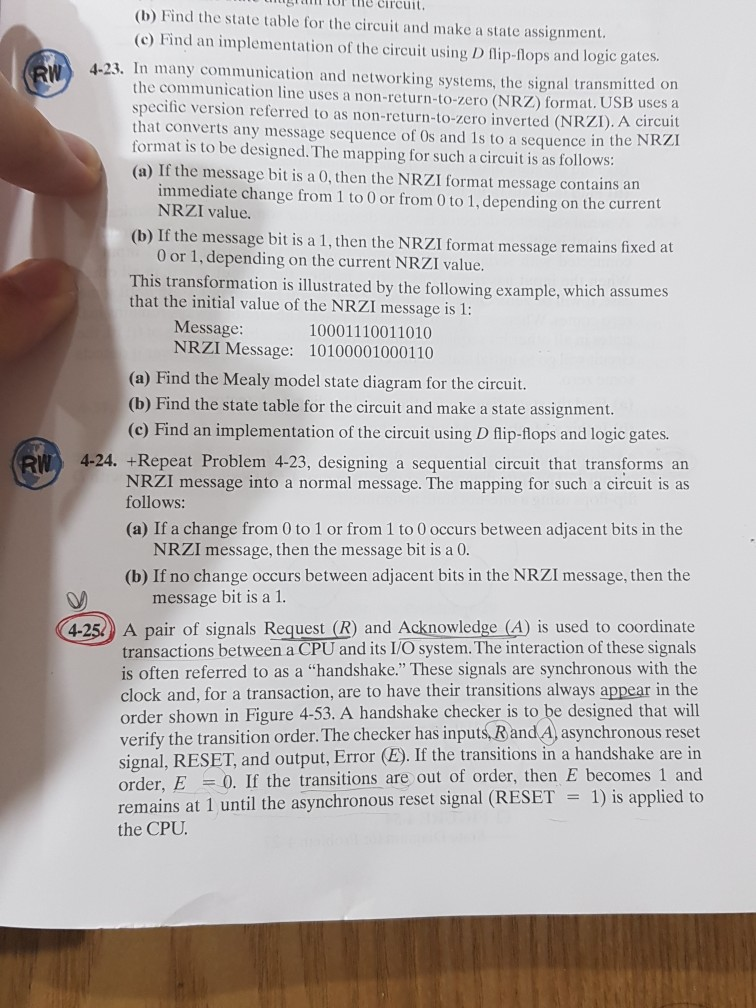how to slove 4-25,26,27 ?? and please 2way slove state assignment gray code and counting Order or tIne Circuit. snTor the (b) Find the state table for the circuit and make a state assignment (c) Find an implementation of the circuit using D flip-flops and logic gates 4-23. In many communication and networking systems, the signal transmitted on the communication line uses a non-return-to-zero (NRZ) format. USB uses a specific version referred to as non-return-to-zero inverted (NRZI). A circuit that...

Free Homework App